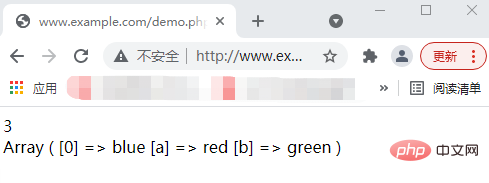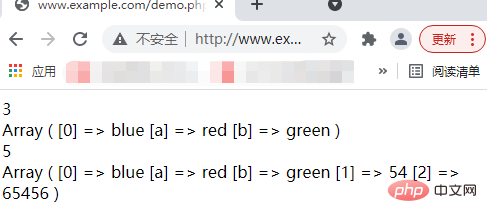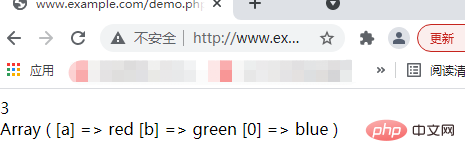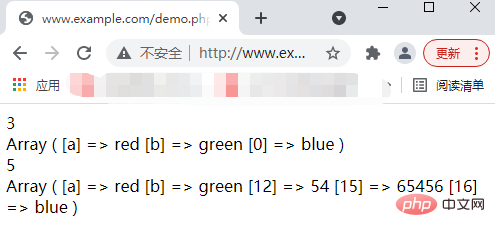# PHP数组学习之怎样在数组的首尾添加元素？`array_unshift`函数-在数组开头添加元素

`array_unshift(array,value1,value2,value3...)`

```<?php
\$a=array("a"=>"red","b"=>"green");
//返回结果的值
print_r(array_unshift(\$a,"blue"));
echo '<br/>';
//添加新元素的新数组
print_r(\$a);
?>``````<?php
\$a=array("a"=>"red","b"=>"green");
//返回结果的值
print_r(array_unshift(\$a,"blue"));
echo '<br/>';
//添加新元素的新数组
print_r(\$a);
echo '<br/>';
\$a1=array("a"=>"red","b"=>"green",12=>"54",15=>"65456");
print_r(array_unshift(\$a1,"blue"));
echo '<br/>';
print_r(\$a1);
?>````array_push`函数-在数组末尾添加元素

`array_push(array,value1,value2...)`

```<?php
\$a=array("a"=>"red","b"=>"green");
//返回结果的值
print_r(array_push(\$a,"blue"));
echo '<br/>';
//添加新元素的新数组
print_r(\$a);
?>``````<?php
\$a=array("a"=>"red","b"=>"green");
//返回结果的值
print_r(array_push(\$a,"blue"));
echo '<br/>';
//添加新元素的新数组
print_r(\$a);
echo '<br/>';
\$a1=array("a"=>"red","b"=>"green",12=>"54",15=>"65456");
print_r(array_push(\$a1,"blue"));
echo '<br/>';
print_r(\$a1);
?>```1/1